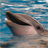QlikView App Dev

Discussion Board for collaboration related to QlikView App Development.

Announcements
QlikWorld 2022, LIVE in Denver CO., May 16-19, 2022. REGISTER NOW TO RECEIVE EARLY BIRD PRICING
cancel
Showing results for
Did you mean:Contributor II

how to create new report from two existing chart expressions in application

Hello All,status A Expression - =MaxString({< [Default Status] = {\$(VcnStatusA)}, Date = {">=\$(=min(StatusA_From_Date))<= \$(=max(StatusA_To_Date))"}>}CN)

Status B Expression =MaxString({< [Default Status] = {\$(VcnStatusB)}, Date = {">=\$(=min(StatusB_From_Date))<= \$(=max(StatusB_To_Date))"}>}CN)

output what i want to display in different report is CN's which fall under difference of date range selected for both the above charts.

2 RepliesLuminary Alumni

Hi,

you can use standard set operations, so you can as well calculate difference between sets:

MaxString({< [Default Status] = {\$(VcnStatusA)}, Date = {">=\$(=min(StatusA_From_Date))<= \$(=max(StatusA_To_Date))"}-{">=\$(=min(StatusB_From_Date))<= \$(=max(StatusB_To_Date))"}>}CN)Contributor II
Author

Thanks Juraj it workedCommunity Browser